# NCERT Solutions For Class 6 Maths Data Handling Exercise 9.4

NCERT Solutions For Class 6 Maths Data Handling Exercise 9.4

### NCERT Solutions For Class 6 Maths Data Handling Exercise 9.4

NCERT Solutions for Class 6 Maths Chapter 9 Data Handling Ex 9.4

Exercise 9.4

Ex 9.4 Class 6 Maths Question 1.
A survey of 120 school students was done to find which activity they prefer to do in their free time.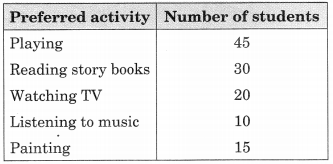Draw a bar graph to illustrate the above data taking scale of 1 unit length = 5 students. Which activity is preferred by most of the students other than playing?
Solution:
From the given information, we have the following bar graph.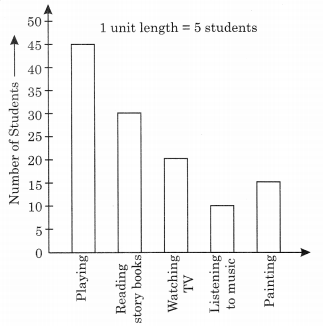Activity reading story books is preferred by the most of the students other than playing.

Ex 9.4 Class 6 Maths Question 2.
The number of Mathematics books sold by a shopkeeper on six consecutive days is shown below: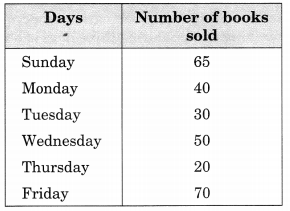Draw a bar graph to represent the above information choosing the scale of your choice.
Solution:
From the given information, we have the following bar graph: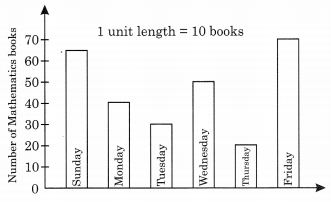Ex 9.4 Class 6 Maths Question 3.
Following table shows the number of bicycles manufactured in a factory during the years 1998 to 2002. Illustrate this data using bar graph. Choose a scale of your choice.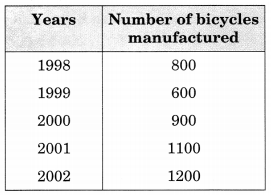(a) In which year were the maximum number of bicycles manufactured?
(b) In which year were the minimum number of bicycles manufactured?
Solution:
From the given information, we have:
(a) In the year of 2002, the maximum number of the bicycles were manufactured.
(b) In the year of 1999, the minimum number of the bicycles were manufactured.Ex 9.4 Class 6 Maths Question 4.
Number of persons in various age groups in a town is given in the following table.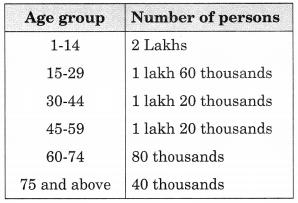Draw a bar graph to represent the above information and answer the following questions, (take 1 unit length = 20 thousands)
(a) Which two age groups have same population?
(b) All persons in the age group of 60 and above are called senior citizens. How many senior citizens are there in the town?
Solution:
From the above information, we have the bar graph.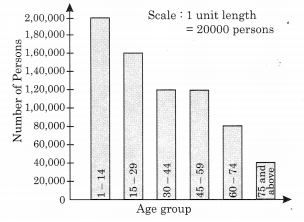(a) Age groups of 30 – 44 and 45 – 59 have the same population.
(b) Number of Senior citizens are 80,000 + 40,000 = 1,20,000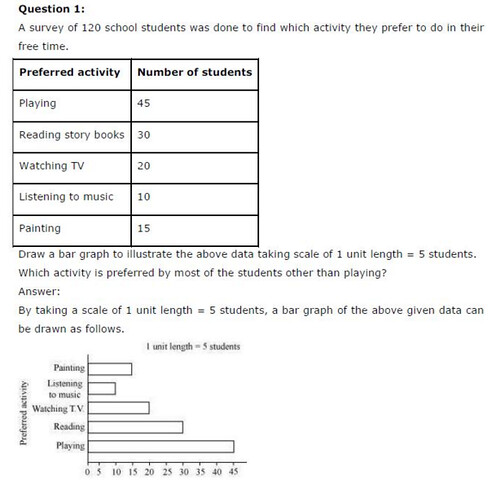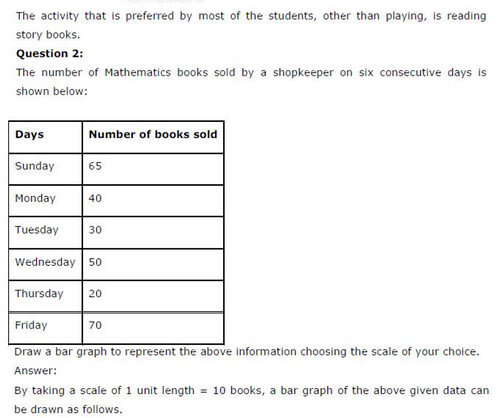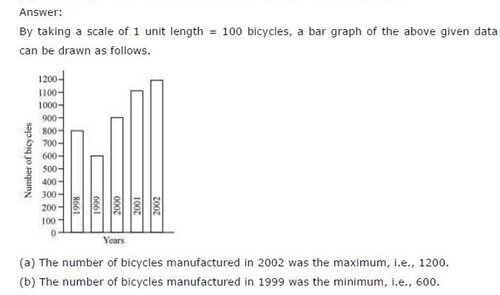## SabDekho

The Complete Educational Website